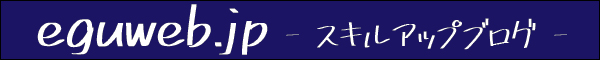# Google Spreadsheet Compound Interest Calculation｜How to perform compound interest calculations (simple version)

In this article, we will introduce compound interest and compound interest calculations.

## What is compound interest?

Compound interest (or compound interest) refers to interest calculated using the compound interest method, whereby interest generated by the principal is incorporated into the principal in the next period and interest is also earned on the interest.

The gradual increase in interest in each period theoretically results in a snowball effect of interest.

## What is simple interest?

Simple interest is when only the initial principal is the source of interest.

Simple interest is when only the initial principal is the source of interest.

Now let’s calculate the compound interest.

## Calculate compound interest in a spreadsheet

Interest is added to the principal in the following year.

In this way, interest is charged on the principal portion of the loan, so the interest earned will be higher than when simple interest is charged.

Your total amount is higher than it was for the simple interest!

## summary

In this issue, we introduced the basics of “compound interest. Compound interest seems to have such amazing power that even the famous Einstein said, “Compound interest is the greatest invention by mankind.

In the next issue, we will discuss other methods of calculating compound interest using the spreadsheet.